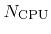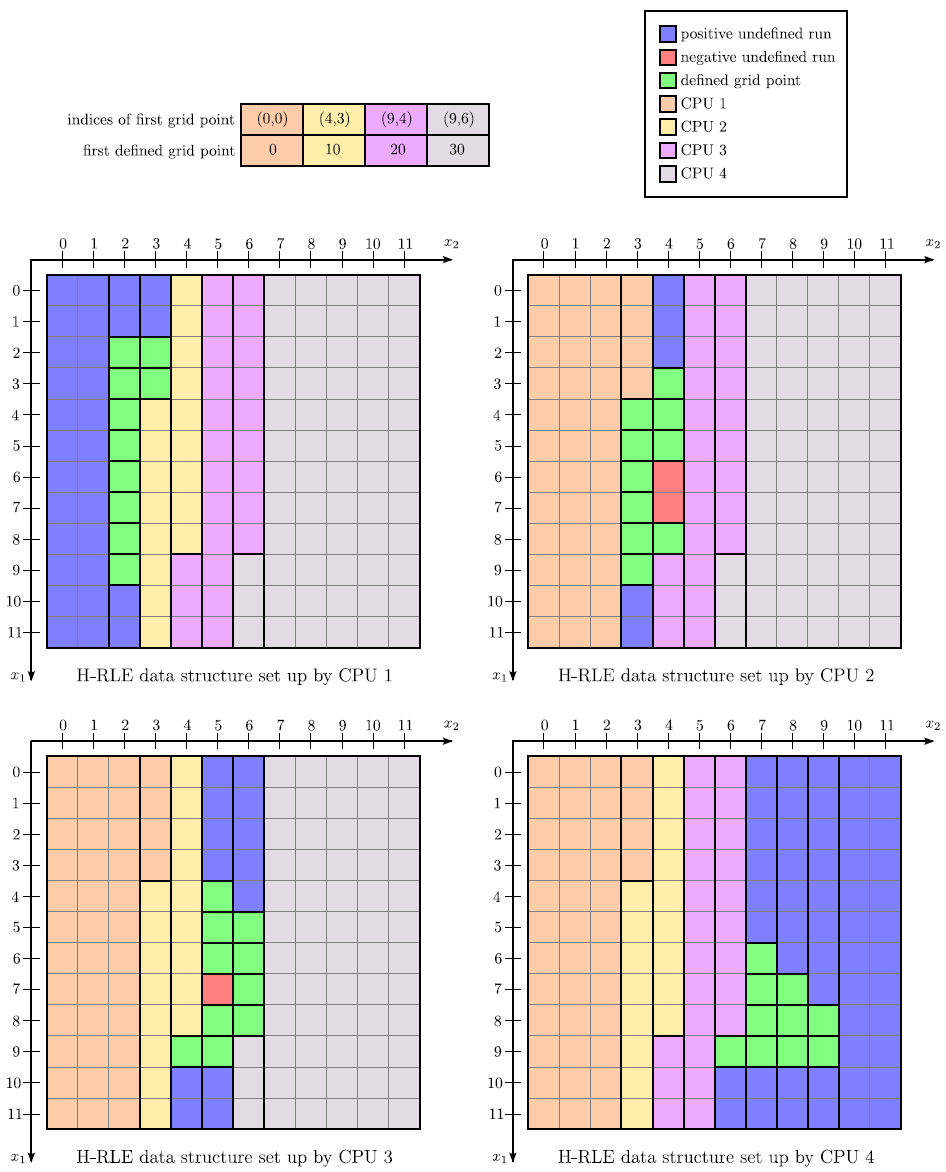Next: 4.10.2 Data Access Up: 4.10 Parallelization Previous: 4.10 Parallelization

## 4.10.1 Parallelization Strategy

In order to achieve a high efficiency, all computational work should be uniformly distributed over all CPUs. According to an idea described in  a good load balance is obtained for the sparse field LS method, if the number of processed active grid points is the same for all CPUs. This can be achieved by analyzing the distribution of active grid points along a certain grid direction and defining slabs of the grid which all contain approximately an equal number of active grid points. If each thread processes one of these slabs, the expected runtimes are similar, and a good parallel efficiency can be obtained.

If a non-static data structure, such as the H-RLE data structure, is used instead of a full grid, parallelization is more complicated. As described previously in Section 4.3 the data structure must be rebuilt several times for every time step. Adding grid points to the data structure is only possible sequentially and in lexicographical order, and hence cannot be accomplished in parallel. A solution of this problem is to use as many H-RLE data structures as CPUs. This way, each thread may add grid points to its own H-RLE data structure at the same time. However, it must be ensured that all grid points are processed by exactly one thread. Therefore, the entire grid needs to be partitioned in advance. In order to maintain all the good properties of the H-RLE data structure, such as the fast sequential access and the small memory requirements, it is advantageous to divide the grid into sequences of grid points which contain approximately an equal number of active or defined grid points.

IfCPUs are used, the partitioning is characterized byindex vectors. Each index vector represents the start of a sequence of consecutive grid points, which is assigned to a thread and which is stored in the corresponding H-RLE data structure. For grid points stored in the H-RLE data structure of another thread, new run codes are introduced. For each CPU a new run code is defined, which provides information which H-RLE data structure houses the corresponding grid points. Figure 4.20 demonstrates the parallelization of the example given in Figure 3.6 and Figure 3.7. There, the LS values and undefined runs are distributed over four separate H-RLE data structures.Next: 4.10.2 Data Access Up: 4.10 Parallelization Previous: 4.10 Parallelization

Otmar Ertl: Numerical Methods for Topography Simulation